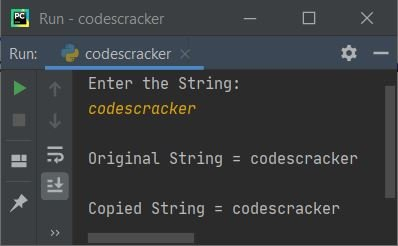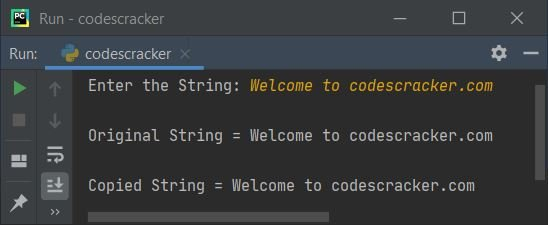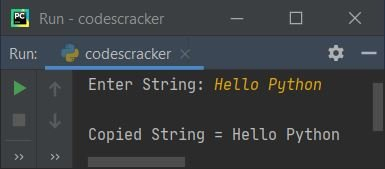# Python Program to Copy String

This article is created to cover some programs in Python, to copy one string to another. Here are the list of approaches used to do the task of copying string entered by user at run-time:

• Copy One String to Another in character by character manner
• Copy String using for loop and indexing
• Using = operator
• Using string slicing

## Copy String Character by Character

To copy one string to another in Python, you have to ask from user to enter a string, then copy that string to another as shown in the program given below. The question is, write a Python program to copy string in character by character manner. Here is its answer:

```print("Enter the String: ")
textOne = input()

textTwo = ""
for x in textOne:
textTwo = textTwo + x

print("\nOriginal String =", textOne)
print("\nCopied String =", textTwo)```

Here is its sample run:Now supply the input say codescracker as string, and then press `ENTER` key to copy the given string into another string, and print the value of both (original and copied) strings as shown in the snapshot given below:In above program, the following block of code:

```for x in textOne:
textTwo = textTwo + x```

states that, each time the character gets copied to x and added with textTwo, then initialized the addition result as new value of textTwo. For example, if user enters codescracker as string, then dry run goes like:

• At first execution of for loop, "c" (first character of textOne) gets initialized to x
• Since x's value (that is "c") is available in textOne. Therefore condition evaluates to be true and program flow goes inside the loop
• Inside the loop, textTwo + x or "" + "c" or "c" gets initialized to textTwo. So textTwo="c"
• Now "o" (second character of textOne) gets initialized to x
• Since x's value (that is "o") is also available in textOne. Therefore condition evaluates to be true again and program flow goes inside the loop
• And inside the loop, textTwo + x or "c" + "o" or "co" gets initialized to textTwo. So textTwo="co"
• This process continues upto the last character of textOne
• In this way, the first string (entered by user) gets copied to second

## Copy String using for Loop and Indexing

This program does the same job as of previous one. But it is created with different approach. This program uses index of entered string to copy its value to another string. Let's have a look at the program first:

```print("Enter the String: ", end="")
sOne = input()

sTwo = ""
for i in range(len(sOne)):
sTwo = sTwo + sOne[i]

print("\nOriginal String =", sOne)
print("\nCopied String =", sTwo)```

Here is its sample run with user input, Welcome to codescracker.com:Note - The end= is used to skip inserting an automatic newline using print().

Note - The len() method returns length of string passed as its argument.

Indexing starts with 0. For example, if user enters a string say codes and this string gets initialized to a variable say sOne. That is, sOne = "codes". Then sOne refers to "c" (first character of "codes"), sOne refers to "o" (second character of "codes"), and so on

Therefore in similar way like done in previous program, this program also copies the string in character by character manner. The dry run of following block of code:

```for i in range(len(sOne)):
sTwo = sTwo + sOne[i]```

with same user input, as provided in above sample run, that is Welcome to codescracker.com goes like:

• Since range() method returns a sequence of values. By default, starts with 0 and increments by 1 every time. Continues until one less than the value passed as its argument.
• Therefore initially i=0 and since 0 is less than len(sOne) or len("Welcome to codescracker.com") or 27, therefore condition evaluates to be true and program flow goes inside the loop
• Inside the loop, sTwo + sOne[i] or "" + sOne or "" + "W" or "W" gets initialized to sTwo
• Similarly, at second evaluation with i's value as 1, sTwo + sOne[i] or sTwo + sOne or "W" + "e" or "We" gets initialized to sTwo
• In this way, I've copied one string to another

## Copy String using = Operator

This is the simplest program to copy one string to another just by using = or initialization operator. Previous two programs are provided for practicing purpose to improve coding skills in Python.

```print("Enter String: ", end="")
sOne = input()

sTwo = sOne
print("\nCopied String =", sTwo)```

Here is its sample run with string input Hello Python:## Copy String using String Slicing

This program does the same job, using string slicing. That is, I've sliced all the characters of entered string by user to another string.

```print("Enter String: ", end="")
sOne = input()

sTwo = sOne[:]
print("\nCopied String =", sTwo)```

Note - While slicing sub-string from a string using [:], empty before : (colon) treated as 0, whereas empty after : (colon) treated as length value of string. Therefore from 0th to last index, all characters gets sliced and copied to sTwo variable.

#### Same Program in Other Languages

Python Online Test

« Previous Program Next Program »